## A tank contains 150 liters of fluid in which 40 grams of salt is dissolved. Brine containing 1 gram of salt per liter is then pumped into th

Question

A tank contains 150 liters of fluid in which 40 grams of salt is dissolved. Brine containing 1 gram of salt per liter is then pumped into the tank at a rate of 3 L/min; the well-mixed solution is pumped out at the same rate. Find the number A(t) of grams of salt in the tank at time t.

in progress 0
4 months 2021-09-05T16:58:32+00:00 1 Answers 59 views 0

The number A(t) of grams of salt in the tank at time t is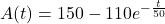Explanation:

Knowing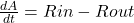First we have to find the Rin and Rout

Rin = (concentration of the salt inflow) * (input rate of brine)

Rin = 1 g/L * 3 L/min = 3 g/L

Rout = (concentration of the salt outflow) * (output rate of brine)

Rout = (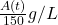) * (3 L/min) =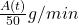Substituting this results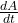= 3 –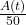–>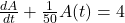Thus, integration factors is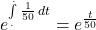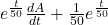A(t) = 4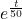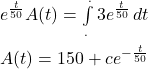Applying the initial conditions

A(0) = 40

c = 150 – 40 = 110

Now, substitute this result in the solution to get